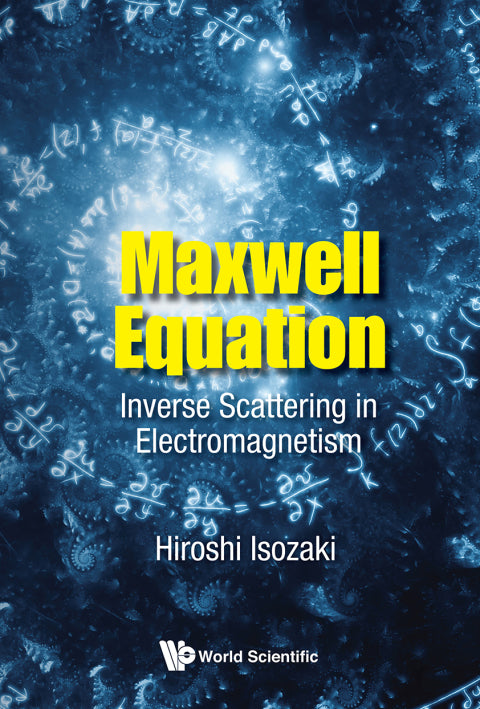• Author(s) Hiroshi Isozaki
• Edition
• Published07122018
• PublisherWorld Scientific Publishing
• ISBN9789813232716
How can one determine the physical properties of the medium or the geometrical properties of the domain by observing electromagnetic waves? To answer this fundamental problem in mathematics and physics, this book leads the reader to the frontier of inverse scattering theory for electromagnetism.The first three chapters, written comprehensively, can be used as a textbook for undergraduate students. Beginning with elementary vector calculus, this book provides fundamental results for wave equations and Helmholtz equations, and summarizes the potential theory. It also explains the cohomology theory in an easy and straightforward way, which is an essential part of electromagnetism related to geometry. It then describes the scattering theory for the Maxwell equation by the time-dependent method and also by the stationary method in a concise, but almost self-contained manner. Based on these preliminary results, the book proceeds to the inverse problem for the Maxwell equation.The chapters for the potential theory and elementary cohomology theory are good introduction to graduate students. The results in the last chapter on the inverse scattering for the medium and the determination of Betti numbers are new, and will give a current scope for the inverse spectral problem on non-compact manifolds. It will be useful for young researchers who are interested in this field and trying to find new problems.

# Maxwell Equation: Inverse Scattering In Electromagnetism

##### Get it instantly

Zookal account needed

\$62.71 \$65.99 Save \$3.28
• Author(s) Hiroshi Isozaki
• Edition
• Published07122018
• PublisherWorld Scientific Publishing
• ISBN9789813232716
How can one determine the physical properties of the medium or the geometrical properties of the domain by observing electromagnetic waves? To answer this fundamental problem in mathematics and physics, this book leads the reader to the frontier of inverse scattering theory for electromagnetism.The first three chapters, written comprehensively, can be used as a textbook for undergraduate students. Beginning with elementary vector calculus, this book provides fundamental results for wave equations and Helmholtz equations, and summarizes the potential theory. It also explains the cohomology theory in an easy and straightforward way, which is an essential part of electromagnetism related to geometry. It then describes the scattering theory for the Maxwell equation by the time-dependent method and also by the stationary method in a concise, but almost self-contained manner. Based on these preliminary results, the book proceeds to the inverse problem for the Maxwell equation.The chapters for the potential theory and elementary cohomology theory are good introduction to graduate students. The results in the last chapter on the inverse scattering for the medium and the determination of Betti numbers are new, and will give a current scope for the inverse spectral problem on non-compact manifolds. It will be useful for young researchers who are interested in this field and trying to find new problems.